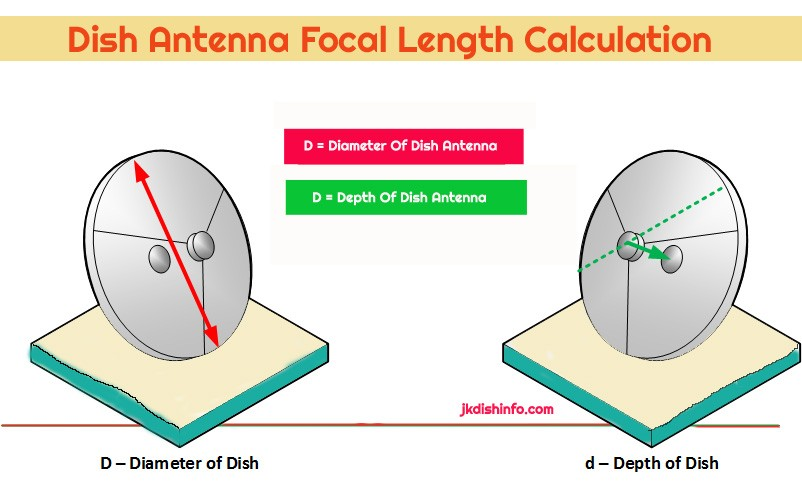# Calculation of focal length of satellite antennaHello friends see this post calculations and find your dish antenna’s perfect focal point.

## Focal Length Calculation of a Satellite Antenna

Focal Length Calculation of a Satellite Antenna

This calculation works for prime focus antennas is useful for checking that the dish is constructed correctly for maximum efficiency and the RF feed horn is in exactly the correct position.

The Focal Length (FL) is the distance from the feed horn to the centre of the antenna.

1. Measure the diameter of the antenna (D) (indicated in red)
2. Measure the depth of the dish (d) (indicated in green). One method is to use some fishing line across the face of the antenna and then measure from the line to the centre of the antenna.
3. Remember to keep all the measurement units the same.
4. Use the below calculation to check the distance from the centre of the surface of the dish to the middle of the RF feed horn.

## Focal Point Calculation

We now look at a more practical situation where we know the dimensions of the dish and we want to find the focal distance which gives the position of the focus relative to the position of the dish as shown in the figure below.

Antenna Focal Distance Calculation D is the diameter of the dish, d is the depth of the dish and f is the focal distance.

#### Focal Length = Diameter / 16 x depth

Which gives a relationship between the diameter D, the depth d and the focal distance f of the dish. The above formula helps in positioning the feed of the parabolic antennas as it gives the focal distance f. Of course in practice the shape of the dish is not a perfect parabola and therefore small adjustments are needed when positioning the feed.

D = Antenna diameter
d = Antenna depth
FL = Antenna focal length (prime focus)

How to find the focal point of a VSAT dish

JK Calculator

## Example:

1 – Find the focal distance f for a dish that has a diameter D = 185 cm and a depth d = 37 cm.
2 – Find a relationship between the diameter D and the depth d so that the focal distance f is equal to twice the depth d of the parabolic dish.
3 – Find a relationship between the diameter D and the depth d so that the focal distance f is greater than d.

error: Content is protected !!
Shopping Cart
Hello
Welcome to The JK Dish Info
We will Reply Soon , Stay tuned
check out: